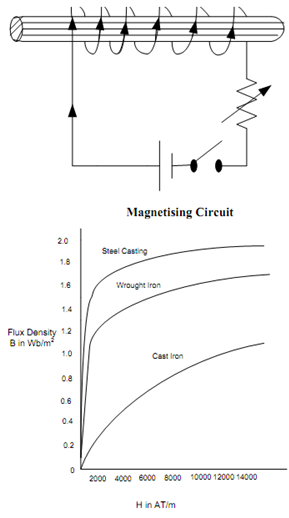## Magnetisation Curve or B-H Curve Assignment Help

Assignment Help: >> ElectroMagnetism - Magnetisation Curve or B-H Curve

Magnetisation Curve or B-H Curve:

If you desire to magnetize a magnetic material like iron, you ought to apply a magnetizing force. This may be done by placing the unmagnetised iron within the field of a solenoid. In Figure we have an electrical circuit which is utilized to magnetise an iron bar AX. If current I in the circuit is raised by varying the variable resistance R, the field H (= NI/l) generated by the solenoid is raised. Correspondingly the flux density B in the iron bar AX is also raised. If flux density B is plotted as a function of field intensity H, we obtain magnetization curve or B-H curve. The approximate magnetization curves of a few magnetic materials are illustrated in FigureMagnetisation Curve or B-H Curve